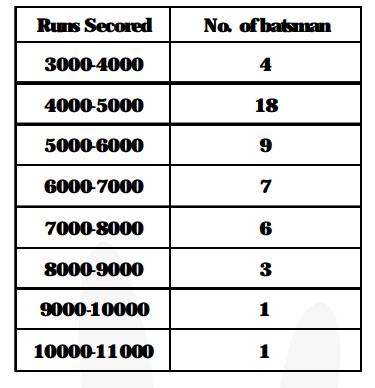# The given distribution shows the number of runs scored by some top batsmen of the world in one day international cricket matches :

Question:

The given distribution shows the number of runs scored by some top batsmen of the world in one day international cricket matches :Find the mode of the data.

Solution:

Modal class = 4000 – 5000

Mode $=\ell+\left\{\frac{\mathbf{f}-\mathbf{f}}{\boldsymbol{2} \mathbf{f}-\mathbf{f}_{\mathbf{0}}-\mathbf{f}_{2}}\right\} \times \mathrm{h}$

$=4000+\left\{\frac{18-4}{2 \times 18-4-9}\right\} \times 1000$

$=4000+\left\{\frac{14}{23}\right\} \times 1000$

= 4608.69